# Analysis on Smoke Visibility in Fire Environment from the Perspectives of Path Curvature and View Direction

Analysis on Smoke Visibility in Fire Environment from the Perspectives of Path Curvature and View Direction
Guangxi Technological College of Machinery and Electricity, Nanning 530007, China

Xi’an University of Science and Technology, Xi’an 710054, China

Corresponding Author Email:
wuwenzhong@tju.edu.cn
Page:
1133-1140
|
DOI:
https://doi.org/10.18280/ijht.370423
23 March 2019
|
Accepted:
2 September 2019
|
Published:
26 December 2019
| Citation

OPEN ACCESS

Abstract:

Besides internal factors like fire growth and its smoke properties, smoke visibility is influenced by external factors like view direction and path curvature. However, the two external factors are not included in the simulation of in Fire Dynamic Simulator (FDS). To make up for the gap, this paper explores the extinction coefficient of smoke based on path curvature, and discusses the affordance of road signs from the perspective of path curvature and view direction, creating a new equation for visibility. The author also compared the visibility values of the new equation and the FDS model. Finally, the new expression of smoke visibility was illustrated through an example of sign design in curved segment. The research results provide a valuable reference for the design of signage system for evacuation in the fire environment.

Keywords:

smoke visibility, path curvature, signage system, view direction, affordance

1. Introduction

Smoke visibility is defined as the maximum distance that an observer can identify the direction sign and emergency sign through the smoke in the fire environment [1-3]. Often evaluated on Fire Dynamics Simulator (FDS), smoke visibility is influenced by external and internal factors [4, 5]. The internal factors are the focus of relevant research, including fire growth and smoke properties like toxicity, opacity and sublethal effect. The smoke properties reduce the visibility and hinder the evacuation in fire environment [6-15].

Kang  pointed out the inconsistency between the above definition and the FDS simulation: the definition indicates that smoke visibility is path-dependent, but the FDS model does not solve the visibility based on the line-of-sight (LOS), and developed a smoke model that evaluates visibility directly as a line integral. Kashef et al.  discussed the difference in smoke visibility between fire scenes in a roadway tunnel, revealing that the fire scenes in curved segment have higher smoke optical density (SOD) and lower mean visibility than those in straight segment. Fu et al. , Lujak et al. , Blin , Gibson  explored the interaction between occupants and signage system in building environment, highlighting the necessity to improve the affordance of current emergency guidance signs. Fujii et al.  carried out two experiments on the visibility of a direction sign and three emergency signs through fire smoke, and derived the expressions of each sign relative to visible distance, smoke density and the acute yaw angles with view direction.

Kashef et al. , Fu et al. , Fujii et al.  also suggested that path curvature has an impact on visibility, and the view direction may affect the affordance of road signs. However, these two external factors of visibility are not included in the FDS modelling. To make up for the gap, this paper explores the extinction coefficient of smoke based on path curvature, and discusses the affordance of road signs from the perspective of path curvature and view direction, creating a new equation for visibility. The author also compared the visibility values of the new equation and the FDS model. Finally, the new expression of smoke visibility was illustrated through an example of sign design in curved segment.

This study discusses smoke’s extinction coefficient with path curvature, and sign’s affordance with path curvature coupled with view direction, and then obtains a new visibility equation. Comparison of visibility values between the new equation and FDS model is done, too. Finally, an application example of the new smoke visibility is illustrated for designing sign in a curved region.

2. Fire Growth and Its Smoke Properties

A turbulent ensemble of combustion reactions might evolve into a fire with the temperature up to 1,500-2,500K, producing energy at 108 W/cm3 per unit volume.

Because few chemical reactions are complete, the combustion in fire is often incomplete under various conditions. Many products of imperfect combustion could be generated, namely, carbon monoxide (CO), hydrogen (H2), hydrogen cyanide (HCN) gas, hydrogen chloride (HCl) gas, and soot, which is predominantly carbon (C). Smoke is produced through the decomposition and combustion of materials in the fire environment. The main components of smoke are different types of particles, ranging from solid fragments, liquid droplets to solid fragments coated with liquid or pitch. The smoke is toxic and corrosive, making the fire zone less visible.

The incompleteness and smoke intensity of a fire reaction are determined by measurement. The yield of a combustion product can be computed by :

$yield\ of\ combustion\ product\ i,\ {{y}_{i}}=\frac{mass\ of\ spcies\ i}{mass\ loss\ of\ sample}$

Taking the fire on a wooden crib for example, the chemical composition of wood was assumed as CH1.7O0.72-0.74N0.000-0.002, and the relevant data were extracted from ASTM E2058-19, Standard Test Methods for Measurement of Material Flammability Using a Fire Propagation Apparatus (FPA) . Then, the combustion reaction of the fire can be expressed as:

\begin{align} & C{{H}_{1.7}}{{O}_{0.74}}{{N}_{0.002}}+1.02121\left( {{O}_{2}}+3.76{{N}_{2}} \right) \\ & \to 0.964679C{{O}_{2}}+0.003655CO+ \\ & 0.848241{{H}_{2}}O+0.035184{{C}_{0.9}}{{H}_{0.1}}+3.838558{{N}_{2}} \\ \end{align}

Thus, the actual yields of combustion products can be obtained as:

${{y}_{CO}}=0.004$, ${{y}_{C{{O}_{2}}}}=1.30$,

$yield\ of\ \text{total gaseous }hydrocarbon,\ {{y}_{HC}}=0.001$,

${{y}_{smoke}}=0.015$.

3. Human Vision and Cognition in Fire Smoke

For human eyes, the wavelength of visible light falls in the range of 380-780 nm. The visual information of brightness, graphs, colors, motions and visibility are acquired based on light intensity, light intensity distribution, wavelength, photo-movement and visible distance, respectively [24, 25].

The direction and emergency signs for evacuation are important tools for fire protection. Familiarity with these signs is critical to the successful evacuation in the fire environment, and must be improved through intense publicity and training. It is a challenge to recognize the information of these signs through fire smoke. In fact, the recognition is under the combined effect of the actual smoke yield of combustion reaction and the emptying rate of the smoke. To cope with the challenge, a multimodal approach could be adopted to recognize the context of each sign, the evacuation behavior and smoke properties (Figures 1-3 ), where Cs is the extinction coefficient.

## 1.jpg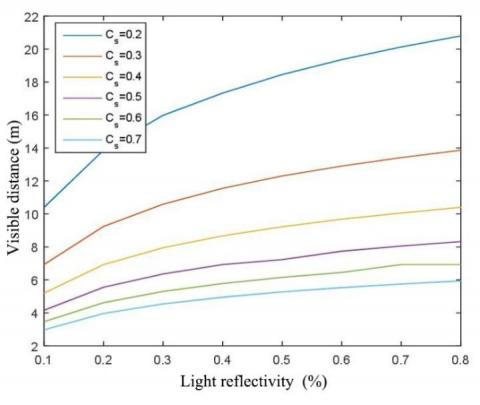Figure 1. Visible distance of light-reflective coating

## 2.jpg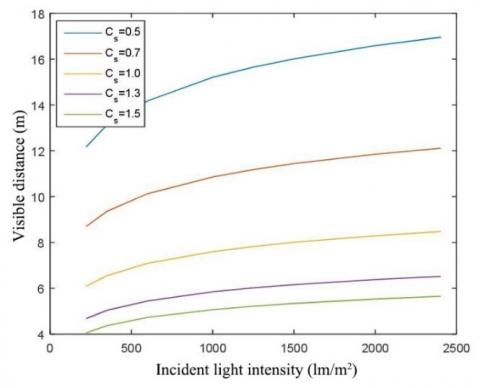Figure 2. Visible distance of self-luminous sign

## 3.jpgFigure 3. Evacuation speed in gases of different properties

As shown in Figures 1-3, the visual distance of light-reflective coating material and self-luminous sign decreases with the growing smoke density; under a special smoke density, the visual distance of light-reflective coating material is positively correlated with its light reflectivity, and that of self-luminous sign has a positive correlation with the incident light intensity; it is easier to evacuate in nonirritant gas than in irritant gas, and the evacuation speed in irritant gas quickly drops to the walking speed of the blind.

4. Extinction Coefficient of Smoke Based on Path Curvature

During the SOD measurement using a smokemeter, the smoke visibility (m) can be calculated by [4, 5]:

$V I S=\frac{C}{C_{s}}$         (1)

where, C falls in 5-10 for a self-luminous sign and 2-4 for a light-reflective sign; Cs is the extinction coefficient.

4.1 Path-dependent extinction coefficient

In FDS modelling, the smoke visibility is simulated by formula (1), and the extinction coefficient is computed based on the composition of the smoke :

$C_{s}=K_{m}\left(\rho_{s} Y_{s}\right)$    (2)

where, Km is the mass specific extinction coefficient (default value: 7,600m2/kg); $\rho_{s} Y_{s}$ is the density of smoke particles (kg/m3).

According to Bouguer’s law [16, 27, 28], the light intensity decreases with the smoke propagation, and the extinction coefficient can be evaluated by :

$\frac{I}{I_{0}}=\exp \left(-C_{s} \times L\right)$        (3)

where, L is the path length of light; I is the emergent light intensity; I0 is the incident light intensity; Cs is the extinction coefficient.

Because the extinction coefficient varies along the LOS , formula (3) can be rewritten to compute the path-dependent extinction coefficient :

$\frac{I}{I_{0}}=\exp \left(-\int_{L} C_{s}(s) d s\right)$       (4)

4.2 Influence of path curvature on extinction coefficient

Kashef et al.  conducted four full-scale fire tests in a tunnel section, which extends from CH 17+00 (east opening of Tube A) to CH45+00 (west opening of Tube C) (Figure 4a). The first two fires were simulated in the straight segment at CH28+50, and another two fires in the curved segment at CH36+00. To minimize the visible smoke and heat release rate (HRR), the fire tests were conducted using a clean combustion propane system (power: 1MW). The ventilation plans for the four tests are listed in Table 1. The test results show that the SODs of the fires in the curved segment were greater than those in the straight segment (Figures 4b-c).

Kashef et al.  also carried out seven FDS simulations for fires at the centerline of the tunnel at CH28+31 and CH36+53. The fire on a 30MW gasoline pool was modelled to simulate a bus or truck on fire. The ventilation plans for the 7 simulations are listed in Table 2. The simulation results show that the fires in the straight segment had better mean visibility than those in the curved segment (Figures 5a-b).

## 4.jpg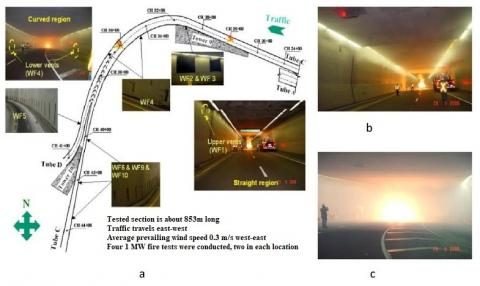Figure 4. (a)The tunnel section; (b) The fire environment in straight segment; (c)The fire environment in curved segment

## 5a.jpg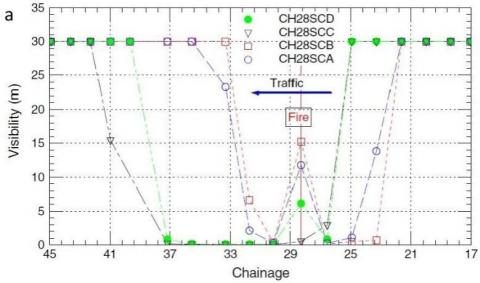## 5b.jpg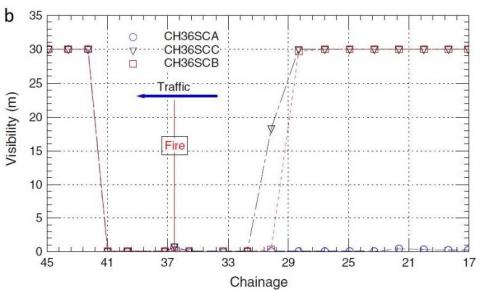Figure 5. Mean visibility at the centerline of the tunnel in fires at (a) CH28+31, (b)CH36+53

Table 1. The ventilation plans for the four fire tests

 Ventilation plans Activated fans Operation mode Total mass flow rate (kg/s) CH28SCA CF1 CF2 WF1 WF2, WF3 Supply Supply Exhaust Exhaust -64.38 0.0 26.48 102.51 CH28SCB CF1 CF2 WF1 WF2, WF3 Exhaust Exhaust Exhaust Exhaust 107.29 80.47 26.48 102.51 CH36SCA WF4 WF6 WF7 WF8 WF5 WF9 Supply Exhaust Exhaust Exhaust Exhaust Exhaust -66.01 31.08 31.52 51.80 38.70 64.45 CH36SCB WF4 WF6 WF7 WF8 WF5 WF9 Exhaust Exhaust Exhaust Exhaust Exhaust Exhaust 39.60 31.08 31.52 51.80 38.70 64.45

Table 2. The ventilation plans for the FDS simulations

 Ventilation plans Activated fans Operation mode Total mass flow rate (kg/s) CH28SCA CF1 CF2 WF1 WF2, WF3 Supply Supply Exhaust Exhaust -64.38 0.0 26.48 102.51 CH28SCB CF1 CF2 WF1 WF2, WF3 Exhaust Exhaust Exhaust Exhaust 107.29 80.47 26.48 102.51 CH28SCC CF1 CF2 WF1 WF2, WF3 CF3, CF4, CF5 Supply Supply Exhaust Exhaust Supply -64.38 0.0 26.48 102.51 -112.84 CH28SCD WF1 WF2, WF3 CF3, CF4, CF5 Exhaust Exhaust Supply 26.48 102.51 -112.84 CH36SCA WF4 WF6 WF7 WF8 WF5 Supply Exhaust Exhaust Exhaust Exhaust -66.01 31.08 31.52 51.80 38.70 CH36SCB WF4 WF6 WF7 WF8 WF10 WF5 Exhaust Exhaust Exhaust Exhaust Exhaust Exhaust 39.60 31.08 31.52 51.80 31.51 38.70 CH36SCC WF4 WF6 WF7 WF8 WF10 WF5 CF3, CF4, CF5 WF2, WF3 Exhaust Exhaust Exhaust Exhaust Exhaust Exhaust Supply Exhaust 39.60 31.08 31.52 51.80 31.51 38.70 -112.84 102.51

4.3 Extinction coefficient based on path curvature

Let s and $\vartheta$ be the arc length and the central angle of a curved segment (Figure 6). Then, the path curvature can be described as $K=\frac{\vartheta}{s}$, and also as $K=\frac{1}{R}$, with R being the radius of the circle of which the arc is a part.

Based on the Kashef et al.  data on the SOD and mean visibility, the extinction coefficient based on path curvature can be expressed as:

$C_{s}(K)=C_{s, c} \times \exp (K)$     (5)

where, Cs,c is extinction coefficient in the straight segment; K is the path curvature in the curved segment.

Formula (5) shows that, if the extinction coefficient in the straight segment is a constant Cs,c, then the extinction coefficient in the curved segment, whose curvature is K, should be multiplied by a large coefficient, at the same fire growth and smoke properties.

## 6.jpg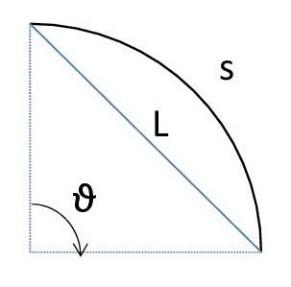Figure 6. The arc length and the central angle of a curved segment

5. Sign Affordance Based on Path Curvature and View Direction

According to the general rules for evacuation, direction and emergency signs must be installed in indoor and building environments.

5.1 Definition of sign affordance

Fu et al. , Lujak et al. , Blin , Gibson  defined the affordance of an object/space and that of a corridor:

The affordance of an object/space refers to an attribute providing the observer/user with insights into its use or purpose. The affordance of a corridor stands for the appearance suggesting that the corridor is a viable egress route.

Drawing on these definitions, the affordance of a sign can be considered as an attribute enabling the observer to recognize the evacuation information of the sign. Obviously, the sign affordance is positively proportional to the C value in formula (1). For simplicity, the affordance of a sign is assumed as equal to C in this research.

5.2 Influence of view direction on sign affordance

## 7.jpgFigure 7. (a) Acute yaw angle of each sign with view direction, (b) Size of direction sign and emergency sign (mm)

Fujii et al.  tested the influence of view direction on the visible distance of signs. The test subjects are a group of 20-year-old college students with visual acuity more than 1.0. The direction and emergency signs (Figure 7) were placed in a 14.8m×14m×2.5m (L×W×H) test room. The acute yaw angle of each sign with view direction and its area perpendicular to view direction are listed in Table 3.

During the tests, the extinction coefficient was adjusted to the preset value based on the SOD collected by a smokemeter. Fujii et al.  derived the expressions of each sign relative to visible distance, smoke density and the acute yaw angles with view direction, indicating that the visible distance of a sign is positively proportional to its area perpendicular to view direction under the specified SOD.

Table 3. The acute yaw angle of each sign with view direction and its area perpendicular to view direction

 $\theta$ (°) 0 30 60 70 80 $B \times L \times \cos \theta$(m2$\times$10-2) 1.69 1.46 0.845 0.578 0.293

5.3 Sign affordance based on view direction

In a curved segment with path curvature K, the acute yaw angle of a sign varies along the view direction (Figure 4a). Let B, L and θ be the height, length and acute yaw angle with view direction of a sign, respectively. Then, the sign affordance has a positive correlation with its area perpendicular to view direction:

$C: B \times L=C^{\prime}: B \times L \times \cos \theta$   (6)

where, C has the same meaning as that in formula (1).

As shown in formula (6), the larger the area of a sign perpendicular to view direction, the better the affordance of the sign. Hence, the sign affordance in the smoke visibility of formula (1) can be modified as:

$C^{\prime}=C \times \cos \theta$        (7)

In a curved segment, the sign may be installed to fit the arc of the curve (Figure 6). Let B and θ be the height and acute yaw angle with view direction of a sign, respectively, and $\vartheta$ be the central angle of the curved segment. Then, the length of the sign can be derived as $S=\frac{1}{K} \times \vartheta$, and the area of the sign perpendicular to view direction can be obtained as:

$A=B \times \frac{1}{K} \times \vartheta \times \cos \theta$           (8)

Hence, formula (7) can be rewritten as:

$C^{\prime}=C \times \frac{1}{L} \times \frac{1}{K} \times \vartheta \times \cos \theta$.                (7)

6. New Equation for Visibility Based on Path Curvature and View Direction

The preceding sections have discussed the extinction coefficient based on path curvature (formula (5)), and examined the sign affordance based on both path curvature and view direction (formula (7)). On this basis, this section devises a new equation for visibility, and compares the visibility of the new equation with that of the FDS model.

6.1 The new equation

Combining formulas (1), (5) and (7), a new equation for smoke visibility can be obtained based on path curvature and view direction:

$V I S_{n e w}=\cos \theta \times \exp (-K) \times \frac{C}{C_{s, c}}$     (9)

where, C has the same meaning as that in formula (1); Cs,c is extinction coefficient in the straight segment; K is the path curvature in the curved segment; $\theta$ is the acute yaw angle with view direction of a sign.

If the sign is installed to fit the arc of a curved segment (Figure 6), formula (9) can be rewritten as:

$V I S_{n e w}=\frac{1}{L} \times \frac{1}{K} \times \vartheta \times \cos \theta \times \exp (-K) \times \frac{C}{C_{s, c}}$    (9)

where, C has the same meaning as that in formula (1); Cs,c is extinction coefficient in the straight segment; K is the path curvature in the curved segment; $\theta$ is the acute yaw angle with view direction of a sign; $\vartheta$ is the central angle of the curved segment; L is the length of the sign.

6.2 Equation verification

The smoke visibility computed by the new equation was compared with the data in Kashef et al.  and Fujii et al. .

(1) Comparison with the smoke visibility in Kashef’s research

Under the prerequisites in Kashef’s research, the parameters were set as: $\theta$=00, C=3, K$\approx$0.02 in straight segment, K=0 in curved segment, Cs=0.21/m. The smoke visibilities of the new equation and Kashef’s research are compared in Table 4 below.

Table 4. Comparison of smoke visibilities of the new equation and Kashef’s research

 Straight segment Curved segment $VIS_{simulated}$ 15m 1m $V I S_{n e w}$ 15m 0.98m $\frac{V I S_{n e w}}{V I S_{s i m u l a t e d}}$ =1 <1

Table 5. Comparison of smoke visibilities of the new equation and Fujii’s research

 Direction sign Emergency sign ${V I S}_{{predicted}}$ 7.727m 11.515m $V I S_{n e w}$ 3.711m 3.711m $\frac{V I S_{n e w}}{V I S_{p r e d i c t e d}}$ <1 <1

As shown in Table 4, the smoke visibility of the new equation was no greater than that simulated. The new visibility is more correct than the simulated, especially in the curved segment, because the FDS simulation ignores the path curvature.

(2) Comparison with the smoke visibility in Fujii’s research

Under the prerequisites in Fujii’s research, the parameters were set as: $\theta$=300, C=3, K=0, Cs=0.71/m (level 1 in Test 1), direction sign B$\times$L=0.148m$\times$0.174m, and emergency sign B$\times$L=0.150m$\times$0.730m. The smoke visibilities of the new equation and Fujii’s research are compared in Table 5 above.

As shown in Table 5, the smoke visibility of the new equation was smaller than that predicted by Fujii. There are two possible reasons for the difference. First, the coefficients in Fujii’s prediction equations are inferred from the test data, which may contain statistical errors that suppress the prediction accuracy. Second, the new equation includes external factors like path curvature and view direction, making the prediction more precise. Of course, more external factors should be considered to further improve the accuracy of the new equation: the emergency sign is longer than the direction sign, but their visibilities predicted by the new equation were the same.

7. Discussion

The new smoke visibility defined in formula (9) is influenced by path curvature, the SOD, the sign affordance, and the acute yaw angle of the sign with view direction.

7.1 Influencing factors of smoke visibility

The interplays between the curvature of light path and the view direction of the sign should be regulated, in order to keep the smoke visibility constant under specified SOD and sign affordance:

(1) In a curved segment, the path curvature K is large, and the acute yaw angle with view direction $\theta$ should be small enough to keep the smoke visibility constant under specified SOD and sign affordance.

(2) In a straight segment, the path curvature K is small, and the acute yaw angle with view direction $\theta$ should be large enough to keep the smoke visibility constant under specified SOD and sign affordance.

7.2 Difference between the smoke visibilities of the new equation and the FDS model

Suppose Cs,c in formula (9) is equal to Cs in formula (4), and the radius of the circle of which the arc is a part is R. If $0 \leq K \leq \frac{1}{R}$ holds for the specified radius of the circle, then $0 \leq \theta \leq \frac{\pi}{2}$. Then, the difference between the new visibility VISnew and that of the FDS model VISFDS can be defined as:

diff$=\left|V I S_{\text {new}}-V I S_{F D S}\right| \geq 0$       (10)

The diff values in the following cases are summarized in Figure 8. Obviously, the new visibility is generally much smaller than that simulated by the FDS model.

Case 1. In a straight segment, K=0, $\theta$=0, diff=0 and VISnew=VISFDS.

Case 2. In a straight segment, K=0, $0<\theta \leq \frac{\pi}{2}$, diff>0 and VISnew<VISFDS for any $\theta$ in $\left(0, \frac{\pi}{2}\right]$.

Case 3. In a curved segment, $0<K \leq \frac{1}{R}$, $\theta$=0, diff>0 and VISnew<VISFDS for any K in $\left(0, \frac{1}{R}\right]$.

Case 4. In a curved segment, $0<K \leq \frac{1}{R}$, $0<\theta \leq \frac{\pi}{2}$, diff>0 and VISnew<VISFDS for any two-tuples $(\theta, K)$ in $\left(0, \frac{\pi}{2}\right] \times\left(0, \frac{1}{R}\right]$.

## 8.jpgFigure 8. The differences in the new visibility and that of the FDS model

8. Application of the New Smoke Visibility

In the light of Figure 8, the ratio between VISnew and VISFDS can be expressed as:

ratio$=\frac{V I S_{\text {new}}}{V I S_{\text {FDS}}} \leq 1$    (11)

If ratio=1 and the sign length is L, then VISnew=VISFDS according to formulas (7), (9) and (11). The result implies the Case 1 above.

If ratio=1 and the sign is installed with a length of s to fit the arc of the curved segment (Figure 6), then VISnew=VISFDS according to formulas (7’), (9’) and (11). The result implies Case 5 above.

Case 5: VISnew=VISFDS, ratio=1, and the interplay between the central angle of the curved segment $\vartheta$ and path curvature K can be restricted by:

$\frac{\vartheta}{L}=K \times \exp (K)$        (12)

where, L is the chord length of sign length s (Figure 6).

Equation (12) implies that, for a specified chord length L of the sign, the sign length s is positively proportional to path curvature K. This correlation provides a concise criterion for the sign design (Figure 9) in curved segments:

$\frac{s}{L}=\exp (K)$        (12)

Because the FDS model ignores view direction and path curvature (formula (1)), the maximum value of the new visibility is achieved in Case 5 (formula 5). To reach this value in a curved segment with path curvature, the sign is usually installed to fit the arc of the curved segment (Figure 6). Figure 9 explains how to design the sign length at a specified path curvature: To obtain the same visibility, the length of a sign with a specified height B must be changed to $s=L \times \exp (K)$ at a curved segment (K>0).

## 9.jpg9. Conclusions

The smoke visibility is not only affected by internal factors like fire growth and its smoke properties, but also influenced by external factors like view direction and path curvature. However, the popular simulation tool for smoke visibility, the FDS, has not included the two external factors into its model. To make up for the gap, this paper discusses the extinction coefficient in fire smoke based on path curvature, and examines sign affordance from the perspectives of path curvature and view direction, creating a new visibility equation. The new equation shows that the two external factors both suppress smoke visibility in a fire zone.

The smoke visibility obtained by the new equation was compared with that simulated by the FDS model. The comparison shows that the new smoke visibility is generally smaller than that of the FDS model. Because the FDS model ignores view direction and path curvature, the maximum value of the new visibility is the visibility in FDS model in case5. To reach this visibility, the direction and emergency signs should be installed to fit the arc of the curve, in a curved segment with path curvature K>0; the length of the signs must be changed to $s=L \times \exp (K)$.

The future research will explore deeper into the visibility in fire smoke. More influencing factors will be introduced to the research, such as the ceiling height.

References

 Hurley, M.J., Gottuk, D.T., Hall Jr, J.R., Harada, K., Kuligowski, E.D., Puchovsky, M., Torero, J.L., Watts Jr , J.M., Wieczorek, C.J. (2015). SFPE Handbook of Fire Protection Engineering. Springer. https://doi.org/10.1007/978-1-4939-2565-0

 Comstock, W.S. (2009). ASHRAE Handbook-Fundamental. Atlanta, GA.

 American Society of Heating. (2007). 2007 ASHRAE Handbook-HVAC Applications (IP. American Society of Heating Refrigerating & Air Conditioning Engineers Incorporated.

 McGrattan, K., Hostikka, S., McDermott, R., Floyd, J., Weinschenk, C., Overholt, K. (2013). Fire dynamics simulator user’s guide. NIST special publication, 1019. http://dx.doi.org/10.6028/NIST.SP.1019

 McGrattan, K., Hostikka, S., McDermott, R., Floyd, J., Weinschenk, C., Overholt, K. (2015). Fire dynamics simulator (FDS Version 6.3.0)-user’s guide. National Institute of Standards and Technology Special Publication, Gaithersburg, MD, 1019.

 Sychta, Z. (1997). Contrast attenuation coefficient as a parameter enabling determination of range of visibility in smoke. Fire and Materials, 21(5): 205-211. https://doi.org/10.1002/(SICI)1099-1018(199709/10)21:5%3C205::AID-FAM609%3E3.0.CO;2-U

 Fridolf, K., Andrée, K., Nilsson, D., Frantzich, H. (2014). The impact of smoke on walking speed. Fire and Materials, 38(7): 744-759. https://doi.org/10.1002/fam.2217

 Löhnert, A., Monreal, N., Knaust, C., Hofmann, A., Krause, U. (2016). CFD modeling approach of smoke toxicity and opacity for flaming and non-flaming combustion processes. Fire and Materials, 40(6): 759-772. https://doi.org/10.1002/fam.2340

 Li, X., Li, D., Yang, X., Yang, J. (2003). Total air age: An extension of the air age concept. Building and Environment, 38(11): 1263-1269. https://doi.org/10.1016/S0360-1323(03)00133-1

 Gann, R.G. (2004). Sublethal effects of fire smoke. Fire Technology, 40(2): 95-99. https://doi.org/10.1023/B:FIRE.0000016898.88522.79

 Butler, K.M., Mulholland, G.W. (2004). Generation and transport of smoke components. Fire Technology, 40(2): 149-176. https://doi.org/10.1023/B:FIRE.0000016841.07530.64

 Wu, W.Z. (2009). Study on air age and smoke-control of fire at subway central-platform. Tianjin: Tianjin University.

 Fourneau, C., Delvosalle, C., Breulet, H., Brohez, S. (2016). Characterization of highly under-ventilated fires using the cone calorimeter. Fire and Materials, 40(3): 434-444. https://doi.org/10.1002/fam.2298

 Lawson, J., Quintiere, J.G. (1986). Slide rule estimates of fire growth. Fire Technology, 22(1): 45-53. https://doi.org/10.1007/BF01040243

 Quintiere, J.G. (1982). Smoke measurements: An assessment of correlations between laboratory and full-scale experiments. Fire and Materials, 6(3-4): 145-160. https://doi.org/10.1002/fam.810060308

 Kang, K. (2007). A smoke model and its application for smoke management in an underground mass transit station. Fire Safety Journal, 42(3): 218-231. https://doi.org/10.1016/j.firesaf.2006.10.003

 Kashef, A., Saber, H.H., Gao, L. (2011). Optimization of emergency ventilation strategies in a roadway tunnel. Fire Technology, 47(4): 1019-1046. https://doi.org/10.1007/s10694-009-0114-8

 Fu, L., Cao, S., Song, W., Fang, J. (2019). The influence of emergency signage on building evacuation behavior: An experimental study. Fire and Materials, 43(1): 22-33. https://doi.org/10.1002/fam.2665

 Lujak, M., Billhardt, H., Dunkel, J., Fernández, A., Hermoso, R., Ossowski, S. (2017). A distributed architecture for real-time evacuation guidance in large smart buildings. Computer Science and Information Systems, 14(1): 257-282. http://dx.doi.org/10.2298/CSIS161014002L

 Blin, F. (2016). The theory of affordances. Language-Learner Computer Interactions, 41-64.

 Gibson, J.J. (2014). The Ecological Approach to Visual Perception. Psychology Press.

 Fujii, K., Sano, T., Ohmiya, Y. (2014). The effect on acute yaw angles with view direction through fire smoke: Visibility of the emergency sign and the direction sign. Journal of Environmental Engineering (Japan), 79(702): 639-648. https://doi.org/10.3130/aije.79.639

 Quintiere, J. (2006). Fundamentals of Fire Phenomena. Wiley.

 Matsuno, S., Sorao, S., Susumu, C., Akehi, K., Mito, K. (2016). Eye-movement measurement for operating a smart device: A small-screen line-of-sight input system. 2016 IEEE Region 10 Conference (TENCON), Singapore. https://doi.org/10.1109/TENCON.2016.7848773

 Marandi, R.Z., Madeleine, P., Omland, Ø., Vuillerme, N., Samani, A. (2018). Eye movement characteristics reflected fatigue development in both young and elderly individuals. Scientific reports, 8(1): 13148. https://doi.org/10.1038/s41598-018-31577-1

 Li, Y.Q. (2007). Performance-based Design in Building Fire Protection Design. Chemical Industry Press.